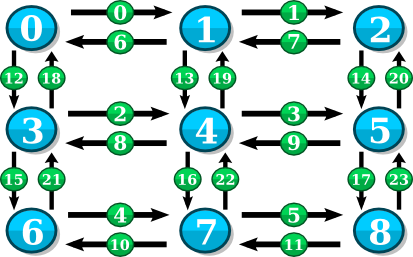#Boost C++ Libraries

...one of the most highly regarded and expertly designed C++ library projects in the world.

# grid_graph

### Overview

A grid_graph represents a multi-dimensional, rectangular grid of vertices with user-defined dimension lengths and wrapping.

grid_graph models:

Defined in boost/graph/grid_graph.hpp with all functions in the boost namespace. All examples are available in a single program file in libs/graph/example/grid_graph_example.cpp

#### Template Parameters

```template <std::size_t Dimensions,
typename VertexIndex = std::size_t,
typename EdgeIndex = VertexIndex>
class grid_graph;
```
• Dimensions - Number of dimensions in the graph, must be greater than 2
• VertexIndex - Type used for vertex indices, defaults to std::size_t
• EdgeIndex - Type used for edge indices, defaults to the same type as VertexIndex

### Creating a Grid Graph

The constructor to grid_graph has several overloads to aid in configuring each dimension:

```// Defines a grid_graph that does not wrap.
grid_graph<...>(boost:array<VertexIndex, Dimensions> dimension_lengths);

// Defines a grid_graph where all dimensions are either wrapped or unwrapped.
grid_graph<...>(boost:array<VertexIndex, Dimensions> dimension_lengths,
bool wrap_all_dimensions);

// Defines a grid_graph where the wrapping for each dimension is specified individually.
grid_graph<...>(boost:array<VertexIndex, Dimensions> dimension_lengths,
boost:array<bool, Dimensions> wrap_dimension);
```

#### Example

```// Define dimension lengths, a 3x3 in this case
boost::array<int, 2> lengths = { { 3, 3 } };

// Create a 3x3 two-dimensional, unwrapped grid graph (Figure 1)
grid_graph<2> graph(lengths);

// Create a 3x3 two-dimensional, wrapped grid graph (Figure 2)
grid_graph<2> graph(lengths, true);

```Figure 1: A 3x3 two-dimensional, unwrapped grid graphFigure 2: A 3x3 two-dimensional, wrapped grid graph

### Indexing

The grid_graph supports addressing vertices and edges by index. The following functions allow you to convert between vertices, edges, and their associated indices:

```typedef grid_graph<...> Graph;
typedef graph_traits<Graph> Traits;

// Get the vertex associated with vertex_index
Traits::vertex_descriptor
vertex(Traits::vertices_size_type vertex_index,
const Graph& graph);

// Get the index associated with vertex
Traits::vertices_size_type
get(boost::vertex_index_t,
const Graph& graph,
Traits::vertex_descriptor vertex);

// Get the edge associated with edge_index
Traits::edge_descriptor
edge_at(Traits::edges_size_type edge_index,
const Graph& graph);

// Get the index associated with edge
Traits::edges_size_type
get(boost::edge_index_t,
const Graph& graph,
Traits::edge_descriptor edge);

// Get the out-edge associated with vertex and out_edge_index
Traits::edge_descriptor
out_edge_at(Traits::vertex_descriptor vertex,
Traits::degree_size_type out_edge_index,
const Graph& graph);

// Get the out-edge associated with vertex and in_edge_index
Traits::edge_descriptor
in_edge_at(Traits::vertex_descriptor vertex,
Traits::degree_size_type in_edge_index,
const Graph& graph);
```

#### Example

```typedef grid_graph<2> Graph;
typedef graph_traits<Graph> Traits;

// Create a 3x3, unwrapped grid_graph (Figure 3)
boost::array<int, 2> lengths = { { 3, 3 } };
Graph graph(lengths);

// Do a round-trip test of the vertex index functions
for (Traits::vertices_size_type v_index = 0;
v_index < num_vertices(graph); ++v_index) {

// The two indices should always be equal
std::cout << "Index of vertex " << v_index << " is " <<
get(boost::vertex_index, graph, vertex(v_index, graph)) << std::endl;

}

// Do a round-trip test of the edge index functions
for (Traits::edges_size_type e_index = 0;
e_index < num_edges(graph); ++e_index) {

// The two indices should always be equal
std::cout << "Index of edge " << e_index << " is " <<
get(boost::edge_index, graph, edge_at(e_index, graph)) << std::endl;

}
```Figure 3: 3x3 unwrapped grid_graph with vertex and edge indices shown.

### Member Functions

There are several grid_graph specific member functions available:

```typedef grid_graph<...> Graph;
typedef graph_traits<Graph> Traits;

// Returns the number of dimensions
std::size_t dimensions();

// Returns the length of a dimension
Traits::vertices_size_type length(std::size_t dimension);

// Returns true if the dimension wraps, false if not
bool wrapped(std::size_t dimension);

// Returns the "next" vertex in a dimension at a given distance. If the dimension
// is unwrapped, next will stop at the last vertex in the dimension.
Traits::vertex_descriptor next(Traits::vertex_descriptor vertex,
std::size_t dimension,
Traits::vertices_size_type distance = 1);

// Returns the "previous" vertex in a dimension at a given distance. If the
// dimension is unwrapped, previous will stop at the beginning vertex in the dimension.
Traits::vertex_descriptor previous(Traits::vertex_descriptor vertex,
std::size_t dimension,
Traits::vertices_size_type distance = 1);
```

#### Example

```typedef grid_graph<3> Graph;
typedef graph_traits<Graph> Traits;

// Define a 3x5x7 grid_graph where the second dimension doesn't wrap
boost::array<std::size_t, 3> lengths = { { 3, 5, 7 } };
boost::array<bool, 3> wrapped = { { true, false, true } };
Graph graph(lengths, wrapped);

// Print number of dimensions
std::cout << graph.dimensions() << std::endl; // prints "3"

// Print dimension lengths (same order as in the lengths array)
std::cout << graph.length(0) << "x" << graph.length(1) <<
"x" << graph.length(2) << std::endl; // prints "3x5x7"

// Print dimension wrapping (W = wrapped, U = unwrapped)
std::cout << graph.wrapped(0) ? "W" : "U" << ", " <<
graph.wrapped(1) ? "W" : "U" << ", " <<
graph.wrapped(2) ? "W" : "U" << std::endl; // prints "W, U, W"

// Define a simple function to print vertices
void print_vertex(Traits::vertex_descriptor vertex_to_print) {
std::cout << "(" << vertex_to_print << ", " << vertex_to_print <<
", " << vertex_to_print << ")" << std::endl;
}

Traits::vertex_descriptor first_vertex = vertex(0, graph);
print_vertex(first_vertex); // prints "(0, 0, 0)"

// Print the next vertex in dimension 0
print_vertex(graph.next(first_vertex, 0)); // prints "(1, 0, 0)"

// Print the next vertex in dimension 1
print_vertex(graph.next(first_vertex, 1)); // prints "(0, 1, 0)"

// Print the 5th next vertex in dimension 2
print_vertex(graph.next(first_vertex, 2, 5)); // prints "(0, 0, 5)"

// Print the previous vertex in dimension 0 (wraps)
print_vertex(graph.previous(first_vertex, 0)); // prints "(2, 0, 0)"

// Print the previous vertex in dimension 1 (doesn't wrap, so it's the same)
print_vertex(graph.previous(first_vertex, 1)); // prints "(0, 0, 0)"

// Print the 20th previous vertex in dimension 2 (wraps around twice)
print_vertex(graph.previous(first_vertex, 2, 20)); // prints "(0, 0, 1)"
```